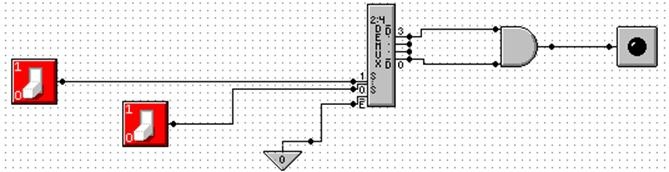Multiplexers and Demultiplexers:

Description and Design Issues

Edward L. Bosworth, Ph.D.

TSYS School of Computer Science

Columbus State University

Columbus, GA 31907

Multiplexers and Demultiplexers

Multiplexer – MUX
Associates One of Many Inputs to a Single Output

Demultiplexer – DEMUX
Associates One Input with One of Many Outputs

Circuit         Inputs      Control          Outputs
Signals

Multiplexer        2N               N                     1
Demultiplexer     1                N                    2N

Sample: 4–to–1 MUX and 1–to–4 DEMUX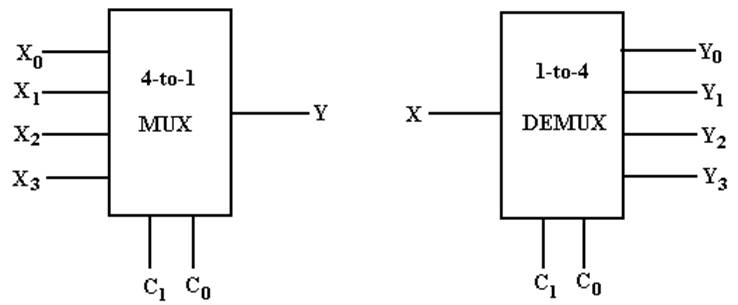My Notation:       X for Input
C for Control Signals
Y for Output

The Multiplexer Equation
Illustrated for a 4–to–1 MUX

Truth table          Denote the multiplexer output by M

 C1 C0 M 0 0 X0 0 1 X1 1 0 X2 1 1 X3

Equation Form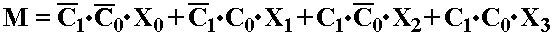Here is another form of the equation that is better when X is used as an input.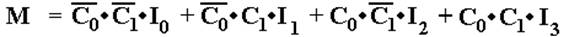Build a 4–to–1 MUX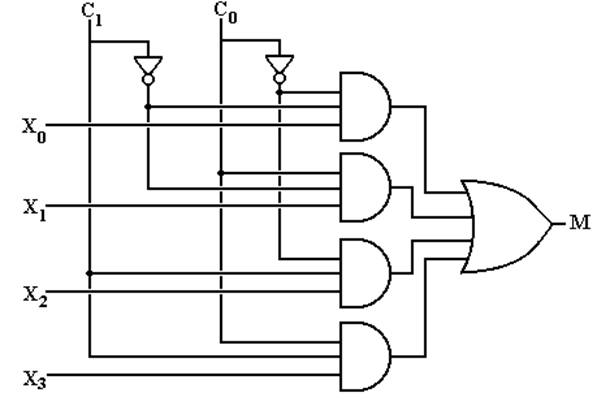But what about an enable input for a multiplexer?

What does it mean for the output of the MUX to be 0?

Multiplexer Attached to a Bus Line

To control a multiplexer’s connection to a common bus, we use a
tri–state buffer and not an enable input to the MUX.  Here I use “E”
as the tri–state control.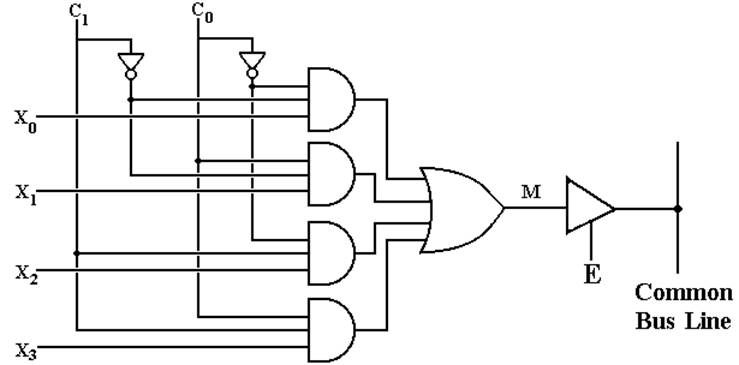When E = 1, the selected MUX input is placed on the bus.
When E = 0, the MUX is detached from the bus; another source feeds the bus.

A 1–to–4 DEMUX

 C1 C0 Selected Output 0 0 Y0 = X Other outputs 0 0 1 Y1 = X Other outputs 0 1 0 Y2 = X Other outputs 0 1 1 Y3 = X Other outputs 0

Build a 1–to–4 DEMUX
With an Enable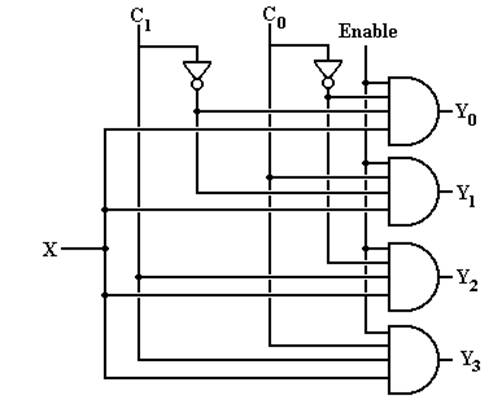If Enable = 0, all outputs are 0.

Using A 2N–to–1 MUX
for a Boolean Function of N Boolean Variables

Theorem 1: Any Boolean function of N Boolean variables, N > 0, can be
constructed by a multiplexer with 2N inputs (usually labeled
IK, IK-1, … I1, I0) and N control lines, labeled CN-1 … C0.

Method:      Express the Boolean function of N Boolean variables in Canonical
Sum of Products and then match the desired function to the
Multiplexer Equation for a 2N–to–1 MUX.

Example:     F2(X, Y, Z) = X·Y + X·Z + Y·Z

Step 1:         This is a function of three Boolean variables.  We must use a
23–to–1 MUX, also called a 8–to–1 MUX.

Using A 2N–to–1 MUX
(page 2)

Step 2:  Convert F2(X, Y, Z) = X·Y + X·Z + Y·Z to Canonical SOP.
Every product term must have a literal for each variable.

A literal is either the variable or its complement.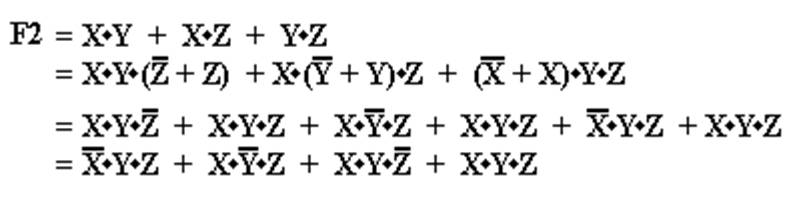Note that all four terms have a literal for each of the three variables X, Y, and Z.

Using A 2N–to–1 MUX
(page 3)

Step 3:  Convert the function to a form with all 2N product terms.
Here we convert F2 to have all eight possible product terms.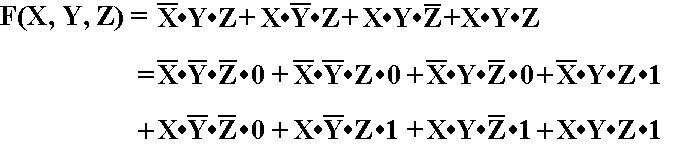Using A 2N–to–1 MUX
(page 4)

Step 4:  Write the Multiplexer Equation for an 8–to–1 MUX.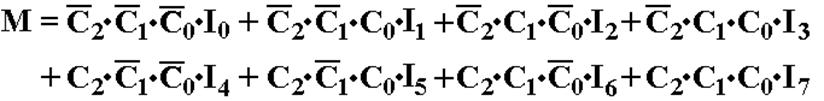Step 5:   Rewrite the equation with C2 = X, C1 = Y, and C0 = Z.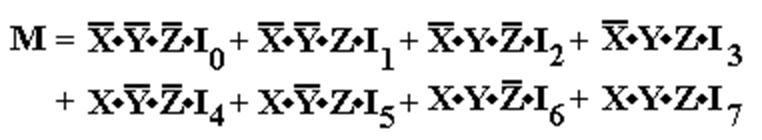NOTE:   Here I use I0, I1, …, I7 as the MUX inputs because I am using X
to denote one of the Boolean variables.

Using A 2N–to–1 MUX
(page 5)

Step 6:  Match the two expressions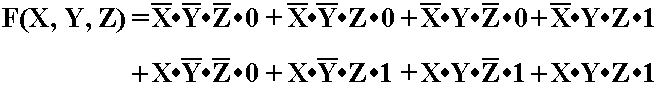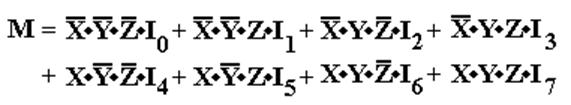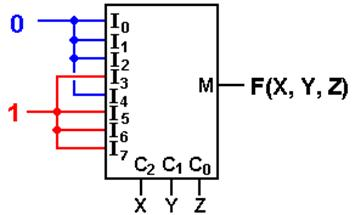I0 = 0     I1 = 0     I2 = 0     I3 = 1
I4 = 0     I5 = 1     I6 = 1     I7 = 1

with C2 = X, C1 = Y, and C0 = Z.

Using A 2N–to–1 MUX
(Using either a
S list or a P list)

For a S list, connect the listed inputs to 1 and the others to 0.

For a P list, connect the listed inputs to 0 and the others to 1.

F(X, Y, Z) = S(3, 5, 6, 7) = P(0, 1, 2, 4)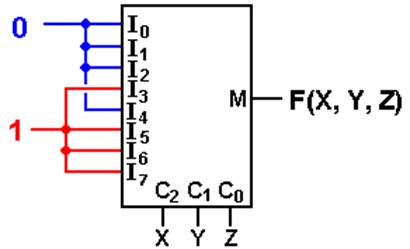We try this with a common circuit emulator, such as Multi–Media Logic,
and find that we need to think a bit more.

An Eight–to–One MUX in Multi–Media

Here is the circuit element selected in the Multi–Media Logic tool.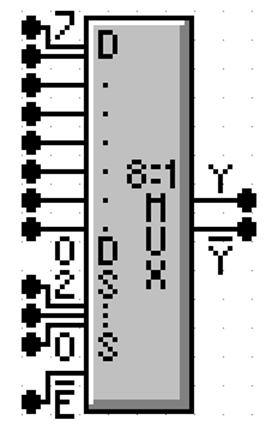This is an 8–to–1 MUX with inputs labeled 7 through 0, or
equivalently X7 through X0.  This is expected.

The selector (control) lines are as expected; 2 through 0.

In my notes, I use M for the output of the Multiplexer.  This
figure uses the symbol Y (not a problem) and notes that real
multiplexers also output the complement.

The only issue here is the enable.  Note that the MUX is
enabled low; this signal must be set to ground in order
for the multiplexer to function as advertised.

Commercial Multiplexer: Enabled and Not Enabled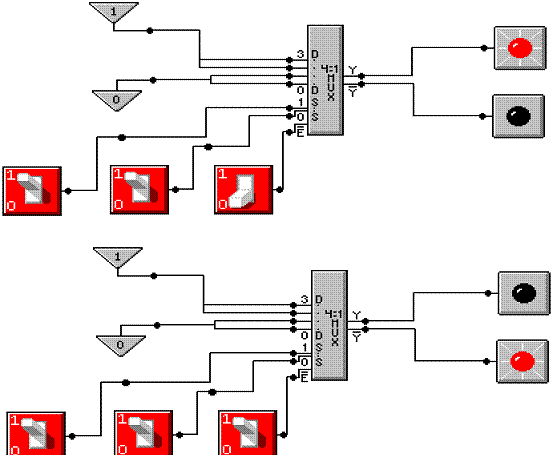At top, the output is X3.  At bottom, the output is 0.

Here is a screen shot of my implementation of F(X, Y, Z) = S(3, 5, 6, 7).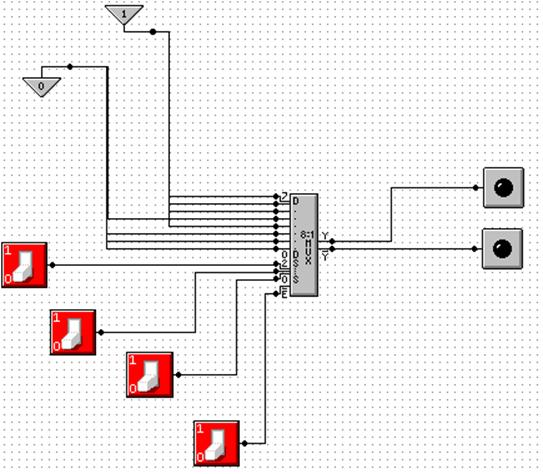NOTE: Show simulation here.

Gray Codes: Minimal Effort Testing

Consider the above circuit with three basic inputs S2, S1, S0.
How can one test all possible inputs with minimum switching?

One good answer is to use Gray Codes for input.  Here are the 2–bit and 3–bit codes.

00                                            000

01                                            001

11                                            011

10                                            010

110

111

101

100

To generate an (N + 1)–bit code set from an N–bit code set.
1.     Write out the N–bit codes with 0 as a prefix, then
2.     Write out the N–bit codes in reverse with 1 as a prefix.

00, 01, 11, 10 becomes 000, 001, 011, 010, 110, 111, 101, and 100

Testing the Carry–Out Circuit

If the Enable switch is set to 1, the output is always 0.  Y’ = 1.

Set the Enable switch to 0 and generate the following sequence.

Start with S2 = 0, S1 = 0, S0 = 0.              0 0 0

Click S0 to get                                         0 0 1

Click S1 to get                                         0 1 1

Click S0 to get                                         0 1 0

Click S2 to get                                         1 1 0

Click S0 to get                                         1 1 1

Click S1 to get                                         1 0 1

Click S0 to get                                         1 0 0

Where are the Decoders?

One will note that the Multi–Media Logic tool does not provide a decoder circuit.

Fortunately, a 1–to–2N demultiplexer can be made into an N–to–2N decoder.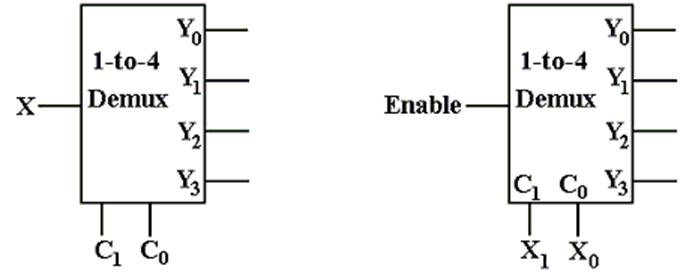Look at the circuit to the left.  The control signals C1,C0 select the output to receive
the input X.  This is exactly equivalent to a decoder.

In the circuit at right, the selected output gets the input, now called “Enable”.
For the demultiplexers we use, the other outputs get a logic 1.

We can fabricate an active low decoder.

The MUX as an Active–Low Decoder

Here is the 2–to–4 Demultiplexer as an 2–to–4 active low decoder.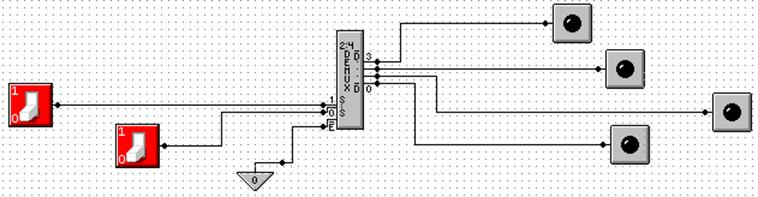Here is an answer to one of the homework problems: use a 2–to–4 decoder for XOR.
The function is either
S(1, 2) or P(0, 3).